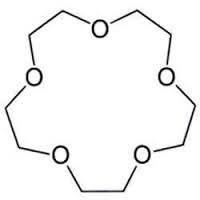# Naming crown ethers

Chemistry Level 1The molecule above is a crown ether. They are named as $\displaystyle{n-\text{crown}-m}$ where $n$ is the total number of atoms (except hydrogen) present in the ring and $m$ is the number of oxygen atoms. For the above crown ether, what is the value of $\displaystyle{m+n}$?

×